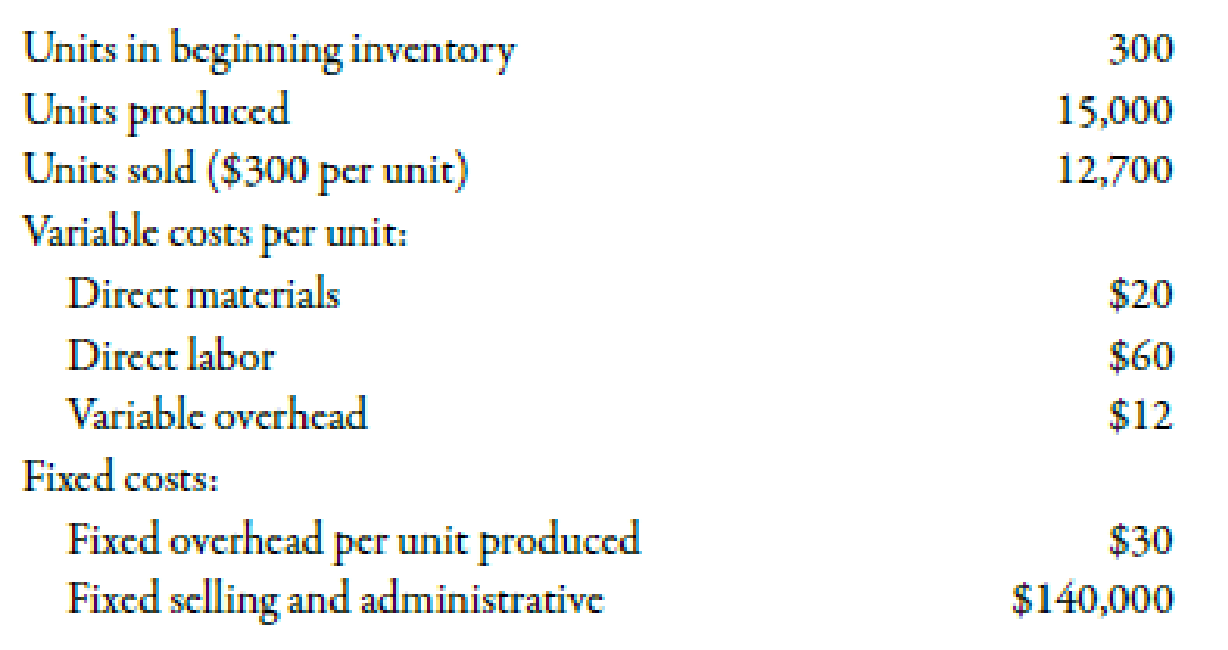Chapter 3, Problem 21BEA### Managerial Accounting: The Corners...

7th Edition
Maryanne M. Mowen + 2 others
ISBN: 9781337115773

#### Solutions

Chapter
Section### Managerial Accounting: The Corners...

7th Edition
Maryanne M. Mowen + 2 others
ISBN: 9781337115773
Textbook Problem
11 views

# Inventory Valuation under Absorption CostingRefer to the data for Judson Company above.Required: 1. How many units are in ending inventory? 2. Using absorption costing, calculate the per-unit product cost. 3. What is the value of ending inventory under absorption costing? Use the following information for Brief Exercises 3-21 and 3-22:During the most recent year, Judson Company had the following data associated with the product it makes:1.

To determine

Calculate the ending inventory units.

Explanation

Cost:

Cost can be defined as the cash and cash equivalent which is incurred against the products or its related services which will benefit the organization in the future. There are two types of costs that are fixed and variable costs.

Use the following formula to calculate the value of ending inventory units:

Ending Inventory=Beggining Inventory+Units ProducedUnits Sold

Substitute 300 units for beginning inventory, 15,000 for units produced and 12,700 for units sold in the above formula

2.

To determine

Compute the per-unit product cost with the help of absorption costing.

3.

To determine

Compute the value of ending inventory with the help of absorption costing.

### Still sussing out bartleby?

Check out a sample textbook solution.

See a sample solution

#### The Solution to Your Study Problems

Bartleby provides explanations to thousands of textbook problems written by our experts, many with advanced degrees!

Get Started

#### Find more solutions based on key concepts